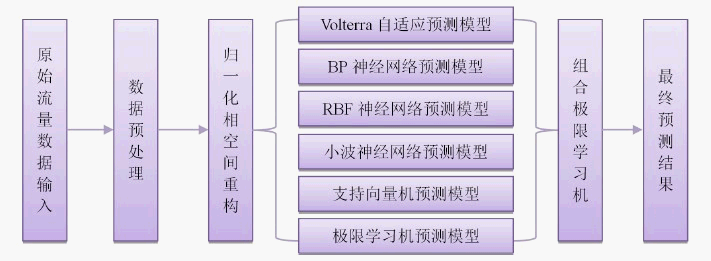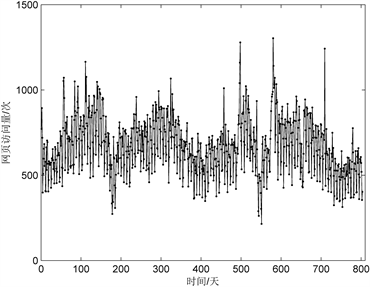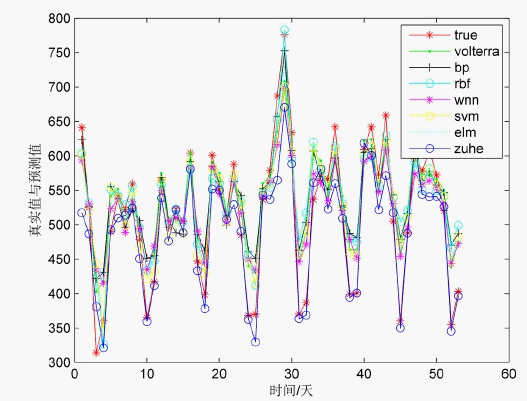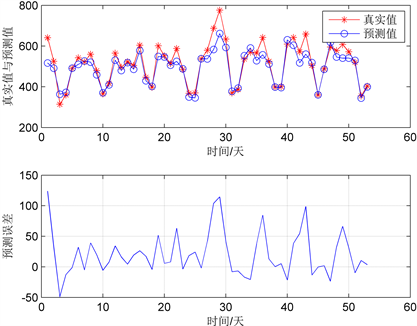﻿ 基于ELM的非线性组合网络流量预测研究

# 基于ELM的非线性组合网络流量预测研究Research on Nonlinear Combined Network Traffic Prediction Based on ELM

Abstract: In order to accurately and reliably predict the short-term trend of network traffic, this paper proposes a nonlinear combination algorithm based on ELM for network traffic prediction based on the chaotic and non-linear characteristics of network traffic. Firstly, the original data are preprocessed, phase space reconstruction, and then Volterra filter adaptive prediction model, BP neural network prediction model, RBF neural network prediction model, wavelet neural network prediction model, SVM prediction model and extreme learning machine prediction model are established. The traffic time series is predicted and analyzed, and then the prediction result is used as the input of the combined ELM to construct a nonlinear combined prediction model. The results show that the mean squared error of the combined forecast is 5.51%, and its evaluation indexes are better than the sub-models. The validity and superiority of the combined strategy are verified, which can provide a better reference for network traffic prediction.

1. 引言

2. 基于ELM的非线性组合预测模型构建

$y=\Phi \left({y}_{1},{y}_{2},\cdots ,{y}_{m},\stackrel{¯}{y}\right)$ (1)

$\underset{j=1}{\overset{L}{\sum }}{\beta }_{i}g\left({W}_{i}\cdot {X}_{j}+{b}_{j}\right)={t}_{j},j=1,2,\cdots ,N$ (2)

Step 1：对于一列原始网络流量时间序列，本文先对其进行数据预处理，利用Q-Q图及标准化残差找出并替换掉其中的异常值。

Step 2：然后对其进行归一化处理，利用C-C法求出嵌入维数m和延迟时间 $\tau$，并在相位空间中对原始数据进行了重构，得到各子模型的训练样本集和测试样本集。

Step 3：然后分别使用Volterra自适应滤波器预测模型、BP神经网络预测模型、RBF神经网络预测模型、小波神经网络预测模型、支持向量机预测模型以及极限学习机预测模型进行独立预测，反复训练网络10次，并取其平均值，得到6个子模型预测结果。

Step 4：将以上6个子模型预测结果及其平均值作为组合极限学习机的输入，反复训练网络10次，并取平均值得到最终的预测结果。Figure 1. Nonlinear combined forecasting model

3. 相空间重构

${S}_{j}=\left(s\left({t}_{j}\right),s\left({t}_{j}+\tau \right),\cdots ,s\left({t}_{j}+\left(m-1\right)\tau \right)\right),\left(j=1,2,\cdots ,M\right)$ (3)

$S={\left[{S}_{1},{S}_{2},\cdots ,{S}_{n}\right]}^{\text{T}}$ (4)

4. 预测子模型构建

4.1. Volterra自适应滤波器预测模型

Volterra是一种非线性自适应FIR滤波器 。由于网络流量的混沌和非线性，Volterra非线性系统函数能够利用本身的高阶矩信息，实现对网络流量的准确预测，本文利用Volterra级数展开公式，建立了网络流量的非线性预测模型，同时由于无穷级数在实际中的实现较为困难，因此本文采用二次截断m次求和，其预测模型为：

$x\left(n+1\right)={h}_{0}+\underset{{i}_{1}=0}{\overset{m-1}{\sum }}{h}_{1}\left({i}_{1}\right)x\left(n-{i}_{1}\tau \right)+\underset{{i}_{1}=0}{\overset{m-1}{\sum }}\underset{{i}_{2}=0}{\overset{m-1}{\sum }}{h}_{k}\left({i}_{1},{i}_{2}\right)x\left(n-{i}_{1}\tau \right)x\left(n-{i}_{2}\tau \right)$ (5)

4.2. BP神经网络预测模型

BP神经网络是一种反向传递并且能够修正误差的多层映射函数，它解决了多层网络中隐含单元学习权的学习问题，能够以任意精度拟合任何连续非线性函数。本文采用三层网络结构：输入层、隐藏层和输出层。其算法主要思想分为信息正向传递和误差反向传播两大过程。本文使用的BP神经网络预测模型  为：

${s}_{i+1}=\frac{1}{1+\mathrm{exp}\left(-\underset{j=1}{\overset{p}{\sum }}{v}_{j}{b}_{j}+\gamma \right)}$ (6)

4.3. RBF神经网络预测模型

RBF神经网络是一种具有多层前向网络类似结构且带有单隐藏层的三层前馈网络  ，它可以任意精度地逼近任意连续函数。构建RBF网络的关键在于构造从输入层到隐藏层 $X\to {\varphi }_{i}\left(X\right)$ 的非线性映射：

${\varphi }_{i}\left(X\right)=\mathrm{exp}\left(\frac{{‖X-{c}_{i}‖}^{2}}{-2{\sigma }_{i}^{2}}\right),\left(i=1,2,\cdots ,m\right)$ (7)

$Y=\underset{i=1}{\overset{m}{\sum }}{\omega }_{i}{\varphi }_{i}\left(X\right)$ (8)

4.4. 小波神经网络预测模型

$f\left(x\right)=\mathrm{exp}\left(-\frac{{x}^{2}}{2}\right)×\mathrm{cos}\left(1.75x\right)$ (9)

$\varphi \left({x}_{i}\right)={f}_{i}\left(\frac{\underset{i=1}{\overset{m}{\sum }}{\omega }_{ij}{x}_{i}-{b}_{j}}{{a}_{j}}\right),j=1,2,\cdots ,p$ (10)

$y=\underset{i=1}{\overset{p}{\sum }}{\omega }_{i}\varphi \left({x}_{i}\right)$ (11)

4.5. 支持向量机预测模型

$K\left(x,y\right)=\mathrm{exp}\left(-\gamma {‖x-y‖}^{2}\right)$ (12)

$f\left(x\right)=\underset{i=1}{\overset{nsv}{\sum }}\left({\alpha }_{i}-{\alpha }_{i}^{*}\right)K\left({x}_{i},{y}_{j}\right)+b$ (13)

5. 模型评价标准

$Err=x\left(n\right)-{x}_{p}\left(n\right)$ (14)

$MAPE=\frac{1}{{N}_{p}}\underset{n=1}{\overset{{N}_{P}}{\sum }}|\frac{x\left(n\right)-{x}_{p}\left(n\right)}{x\left(n\right)}|$ (15)

$Perr=\frac{\underset{n=1}{\overset{{N}_{p}}{\sum }}{\left(x\left(n\right)-{x}_{p}\left(n\right)\right)}^{2}}{\underset{n=1}{\overset{{N}_{p}}{\sum }}{x}^{2}\left(n\right)}$ (16)

6. 实证分析

6.1. 实验环境及数据来源

6.2. 数据预处理Figure 2. Network traffic data (comparison of original data before processing and data after processing)

6.3. 预测结果及分析

${x}^{\prime }\left(i\right)=\frac{x\left(i\right)-\mathrm{min}\left(x\left(i\right)\right)}{\mathrm{max}\left(x\left(i\right)\right)-\mathrm{min}\left(x\left(i\right)\right)}$ (17)

6.3.1. 组合预测结果Figure 3. Comparison between single forecast and combined forecastFigure 4. Combined prediction results and errors

6.3.2. 结果分析

1) 从图3可以看出，6种子模型都具有较高的预测精度，均有预测值与真实值相等的情况存在，能够很好地反映网络流量变化的趋势和规律，而且预测误差也在比较小的误差范围内，从表1可以看出，6种子模型的均方百分误差分别为8.31%，9.46%，8.37%，8.42%，7.86%，7.88%都满足小于10%的预测精度，说明了子模型对网络流量时间序列信息的捕捉比较全面，具有很好的非线性拟合能力，同时进一步说明了这些子模型的有效性与优越性，这也为组合预测打好了良好的基础。

2) 从图4可以看出，组合预测结果已经基本与真实值走势吻合，其预测均方百分误差为5.51%，小于各子模型的误差，说明了本文的组合策略能够较好的综合各子模型所捕捉的序列信息，使得各子模型优势互补，因而得到一个更为精确的预测结果，也说明了本文的组合策略的有效性与可行性，可以为网络流量的预测提供一个较好的参考。Table 1. Error comparison of prediction results

NOTES

*通讯作者。

 顾兆军, 李冰, 刘涛. 基于PSO-Elman模型的网络流量预测[J]. 现代电子技术, 2019, 42(1): 82-86.

 殷礼胜, 何怡刚, 董学平, 等. 交通流量VNNTF神经网络模型多步预测研究[J]. 自动化学报, 2014(9): 2067-2073.

 任师涛, 史志才, 吴飞, 等. 基于改进BP神经网络的路由器流量预测方法[J]. 传感器与微系统, 2018, 37(8): 49-50.

 刘杰, 黄亚楼. 基于BP神经网络的非线性网络流量预测[J]. 计算机应用, 2007(7): 1770-1772.

 王俊松, 高志伟. 基于RBF神经网络的网络流量建模及预测[J]. 计算机工程与应用, 2008, 44(13): 6-7.

 张玉梅, 曲仕茹, 温凯歌. 基于混沌和RBF神经网络的短时交通流量预测[J]. 系统工程, 2007(11): 26-30.

 李欣, 孙珊珊. 基于小波神经网络的网络流量预测研究[J]. 现代电子技术, 2016, 39(23): 98-99.

 雷霆, 余镇危. 一种网络流量预测的小波神经网络模型[J]. 计算机应用, 2006(3): 526-528.

 熊凡. 遗传算法优化支持向量机的网络流量混沌预测[J]. 现代电子技术, 2018, 41(18): 166-169.

 袁开银, 魏彬. 相空间重构和极限学习机的网络流量预测模型[J]. 控制工程, 2018, 25(11): 2087-2091.

 王思哲. 葡萄酒品尝评分的可信性度量方法研究[J]. 海南热带海洋学院学报, 2018, 25(5): 74-79.

 王思哲, 王志刚, 何勇. 基于数据挖掘技术的葡萄酒评价体系研究[J]. 应用数学进展, 2015, 4(4): 376-384.

 Liu, J. and Guo, Z.H. (2014) Network Traffic Prediction Using Radial Basis Function Neural Network Optimized by Ant Colony Algorithm. Sensors & Transducers, 172, 224-228.

 Tan, X.L., Fang, W.J. and Qu, Y. (2013) Network Traffic Prediction Algorithm Based on Wavelet Transform. International Journal of Advancements in Computing Technology, 5, 183-190.

 凌立文, 张大斌. 组合预测模型构建方法及其应用研究综述[J]. 统计与决策, 2019, 35(1): 18-23.

 Ahmad, Z. and Zhang, J. (2008) Selective Combination of Multiple Neural Networks for Improving Model Prediction in Nonlinear Systems Modelling through Forward Selection and Backward Elimination. Neurocomputing, 72, 1198-1204.
https://doi.org/10.1016/j.neucom.2008.02.005

 Yang, Y.M. and Jonathan Wu, Q.M. (2016) Extreme Learning Machine with Subnetwork Hidden Nodes for Regression and Classification. IEEE Transactions on Cybernetics, 46, 2885-2898.
https://doi.org/10.1109/TCYB.2015.2492468

 陈铿, 韩伯棠. 混沌时间序列分析中的相空间重构技术综述[J]. 计算机科学, 2005(4): 67-70.

 雷绍兰, 孙才新, 周湶, 张晓星. 电力短期负荷的多变量时间序列线性回归预测方法研究[J]. 中国电机工程学报, 2006(2): 25-29.

 王兰, 李华强, 吴星等. 基于改进局域Volterra自适应滤波器的风电功率混沌时间序列预测模型[J]. 电力自动化设备, 2016, 36(8): 40-44.

 刘春. 遗传算法优化BP神经网络的网络流量预测[J]. 信息安全与技术, 2014, 5(6): 82-86.

 李立华. 基于径向基函数(RBF)神经网络模型的金融混沌预警研究[J]. 海南金融, 2012(6): 32-35.

Top